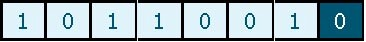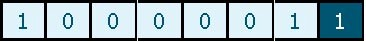# Checking Data

Data stored on a computer is only useful as long as it is correct and up-to-date. It is important to check data when it is entered to make sure that it is both sensible and correct.

If data is not checked before it is processed any errors could cause the final output to be nonsense. Two methods can be used to check data when it is input. These are called verification and validation.

ntent -->

## Verification

• Verification tests to ensure the right entry of results.
• Checking is mostly achieved by making two people access the same data collection on separate machines.
• Once the data are input all users compare the two data sets to validate that it fits.
• Any data not balanced shall be denied.
• Software that may, for example, require that the same data be entered twice could also perform verification.
• If the data does not balance the two entries, it is declined.

## Validation

• The program shall conduct validation tests to ensure that the entered data is appropriate and sensitive.
• Computers reject data that is not sensitive or authorized.
• Validation tests of various kinds may be performed on data by machines.
• This video contains information on checking and validity

## Range Check

Range checks are used to check that data is within a certain range of numbers or a specific set of values.

For example, if the examination marks for a group of students were being inputted a range check could be used to make sure that each mark was greater than or equal to zero and less than or equal to the maximum possible mark.

## Type Check

Type checks are used to check that the correct type of data has been entered in a field.

For example, if numeric data is being inputted a type check could be used to make sure that text data isn’t entered by accident.

## Length Check

Length checks are used to check that input data contains a certain number of characters.

For example, if a value in a certain field had to contain five digits and only four digits were input, an error message would be given to the user.

## Presence Check

A presence check is used to make sure that a value has been entered in a field.

In some database files entering data in certain fields can be optional. Other fields, such as key fields, for example, are compulsory and must have values entered in them.

A presence check makes sure that data is present in a field where a value must be needed.

## Parity check

Sometimes when data is being transferred electronically from one place to another it can become corrupted.

A parity check is used to make sure that data has not been corrupted during transmission.

Data is transmitted as a binary pattern of 0s and 1s.

A parity check involves adding an extra 0 or 1, called a parity bit, to the binary pattern so that the total number of 1s in the pattern is either an even number, this is called even parity, or an odd number, this is called odd parity.

## Even ParityIn even parity, the parity bit is set to either 0 or 1 so that the total number of 1s adds up to an even number.

In this example, there are four 1s so the value 0 is needed in the parity bit to keep the number of 1s even.

## Odd ParityIn odd parity, the parity bit is set to either 0 or 1 so that the total number of 1s adds up to an odd number.

In this example, there are two 1s so the value 1 is needed in the parity bit to make the number of 1s odd.

## Hash total

Hash totals are used to check that groups of numbers have been input correctly.

A hash total is the sum of a group of numbers that are going to be input.

The hash total is input along with the numbers. The computer calculates a hash total for the numbers that have been input.

If the hash total calculated by the computer does not match the hash total that was input with the numbers then one or more of the numbers have either not been entered or have been entered incorrectly.

## Check Digit

Check digits are used to validate long numbers that have a lot of digits in them.

A check digit is an extra digit placed at the end of a long number that can be used to check if the number has been input correctly.

Check digits are often used to check numbers that have been input using direct data entry devices such as bar code scanners or light pens.

The value of a check digit is worked out by performing a calculation using the individual digits that make up a number. This calculation gives the value of the check digit which is then added as an extra digit to the end of the number.

## Calculating check digits using the modulus-11 method

Each digit is assigned a weight starting at 2 with the right-hand digit;

Each digit is multiplied by its weight;

The results of these calculations are added together to give a total;

The total is divided by 11;

The remainder is subtracted from 11 to give the check digit. The two exceptions are: If the remainder is 0 and the result is 11 the check digit is 0, not 11. If the remainder is 1 and the result is 10 the check digit is X, not 10.

## Coding data

When data is input using a manual input device such as a keyboard, errors often occur due to values being entered incorrectly.

A common mistake is to swap two letters or digits around; this is called a transposition error.

One method that can be used to cut down on errors like this is to use coded values for data.

Suppose that a field could contain one of three possible values; small, medium, or large. Instead of typing in the full word each time we could instead type S, M, or L.

## The advantages of coding values

Fewer key presses are needed when entering a value in the field so there is less chance of the wrong keys being pressed;

Time is saved when entering data because there is less to type in each time;

Database packages allow automatic validation checks to be set up to make sure that only the allowed codes have been input in a field.

#### Related Posts